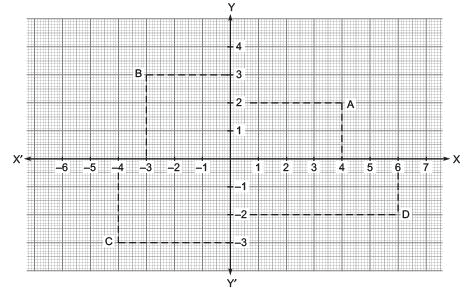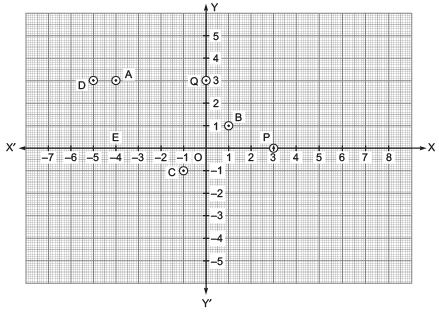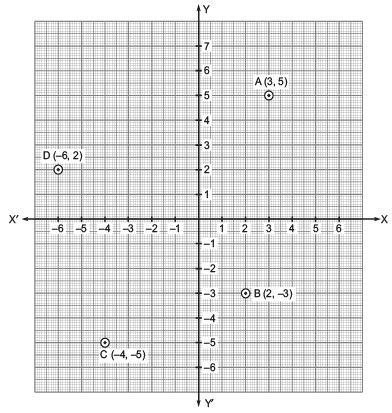# Additional Questions Solutions - Coordinate Geometry Notes | Study Mathematics (Maths) Class 9 - Class 9

## Class 9: Additional Questions Solutions - Coordinate Geometry Notes | Study Mathematics (Maths) Class 9 - Class 9

The document Additional Questions Solutions - Coordinate Geometry Notes | Study Mathematics (Maths) Class 9 - Class 9 is a part of the Class 9 Course Mathematics (Maths) Class 9.
All you need of Class 9 at this link: Class 9

Question 1. Look at the following figure and fill in the blanks:
(i) The abscissa and the ordinate of the point B are ____ and ____ respectively. Hence, the coordinates of B are (____, ____).
(ii) The x-coordinate and the y-coordiante of the point A are ____ and ____ respectively.
Hence, the coordinates of A are (____, ____).
(iii) The x-coordinate and the y-coordinate of the point D are ____ and ____ respectively.
Hence, the coordinates of D are (____, ____).Solution: (i) The abscissa and the ordinate of the point B are –3 and 3 respectively. Hence, the coordinates of B are (–3, 3).
(ii) The x-coordinate and the y-coordinate of the point A are 4 and 2 respectively. Hence the coordinates of A are (4, 2).
(iii) The x-coordinate and y-coordiante of the point D are 6 and –2 respectively. Hence, the coordinates of D are (6, –2).

Question 2. Look at the following figure and answer the following:
(i) What are the coordinates of the origin?
(ii) What is the abscissa of P?
(iii) What is the ordinate of Q?
(iv) What is the x-coordinate of A?
(v) What is the y-coordiante of B?
(vi) Name the point determined by the coordiantes (–5, 3).
(vii) Name the point determined by the coordinates (–1, –1).
(viii) Name the point determined by the coordinates (–4, 0)Solution: (i) The coordinates of origin are (0, 0).
(ii) The abscissa of P is 3.
(iii) The ordinate of Q is 3.
(iv) The x-coordinate of A is –4.
(v) The y-coordinate of B is 1.
(vi) The point D is determined by (–5, 3).
(vii) The point C is determined by (–1, –1).
(viii) The point E is determined by (–4, 0).

Question 3. Draw X'OX and YOY' as axes on the plane of a paper and plot the points given below: (i) A(3, 5) (ii) B(2, –3) (iii) C(–4, –5) (iv) D(–6, 2)
Solution: We draw X'OX and YOY' as axes and fix convenient units. Starting from O, mark equal distances on OX, OX', OY and OY'. Now,(i) Starting from O, take (+3) units on the x-axis and then (+5) units on the y-axis to obtain the point A(3, 5).
(ii) Starting from 0, take (+2) units on the x-axis and then (–3) units on the y-axis to obtain B(2, –3).
(iii) Starting from 0, take (–4) units on the x-axis and then (–5) units on the y-axis to obtain C(–4, –5).
(iv) Starting from 0, take (–6) units on the x-axis and then (+2) units on the y-axis to obtain the point D(–6, 2).

Question 4. In which quadrants do the following points lie?
(i) (5, –3)
(ii) (–8, 4)
(iii) (–3, –6)
(iv) (5, 4)
Solution: (i) ∵ Points of type (+, –) lie in the 4th quadrant.
∴ Point (5, –3) lies in the quadrant IV.

(ii) ∵ Points of the type (–, +) lie in the 2nd quadrant.
∴ The point (–8, 4) lies in the quadrant II.

(iii) ∵ The points of the type (–, –) lie in the 3rd quadrant.
∴ The point (–3, –6) lies in the quadrant III.

(iv) ∵ The points of the type (+, +) lie in the 1st quadrant.
∴ The point (5, 4) lies in the quadrant I.

The document Additional Questions Solutions - Coordinate Geometry Notes | Study Mathematics (Maths) Class 9 - Class 9 is a part of the Class 9 Course Mathematics (Maths) Class 9.
All you need of Class 9 at this link: Class 9Use Code STAYHOME200 and get INR 200 additional OFF

## Mathematics (Maths) Class 9

84 videos|352 docs|109 tests

Track your progress, build streaks, highlight & save important lessons and more!

,

,

,

,

,

,

,

,

,

,

,

,

,

,

,

,

,

,

,

,

,

;